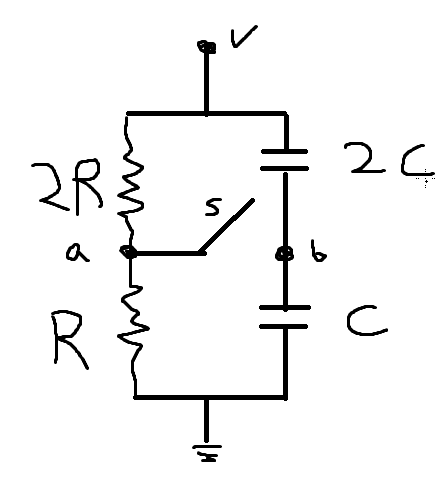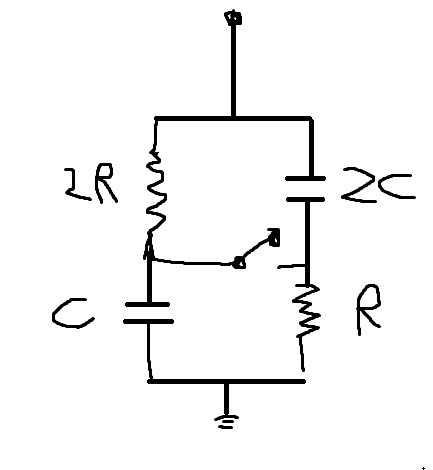# RC circuit and voltage

oreosama

## Homework StatementGiven V R C for the figure, determine

a.
The voltage of a with respect to b when the switch is open?

b.
Which point a or b is at a the higher voltage?

c.
What is the final voltage of point b with respect to ground when the switch is closed?

d.
Wow much does the charge on each capacitor change when the switch s is closed?

## Homework Equations

V = IR
V = q/C

charging conductor
$$q = CE(1-e^{\frac{-t}{RC}})$$
$$i = \frac{E}{R}e^{\frac{-t}{RC}})$$

discharging conductor
$$q = Q_0e^{\frac{-t}{RC}}$$
$$i = I_0e^{\frac{-t}{RC}}$$

## The Attempt at a Solution

a.
I don't really understand conceptually whats going on, so i'll talk through.. the switch has been open, so the conductor takes all of the charge. getting a is solving the righthalf like a simple series capacitor problem to get voltage at b, voltage at a is just V because 0 current over resister means full voltage reaches a

b.
a would be at higher voltage because it has the maximum possible, b has less because the first capacitor takes some away

c.
At this point I feel like im surely conceptually wrong, but if you open the switch, the conductors are still going to be taking all of the current and nothing should be changing ?

d.

i would think none given above

I have some hitches in the concepts going on I guess. If anyone can clarify what is going on I'm sure I can get this quite easily. I didn't have much a problem when resisters/conductors werent together

Homework Helper
Gold Member
Qa. Start by assuming that at some point the power supply has been off and the capacitors are discharged. Assume the switch is allways open (eg delete it from the drawing). Now the power supply is turned on. The circuit simplifies to two separate circuits connected to the supply. Lets call these left and right.

The left hand circuit is just a resistor network - use the potential divider rule to calculate the voltage on point "a" (with respect to earth/0V).

The right hand circuit is a series capacitor network. When the supply voltage was turned on the same current (charge) flowed through both capacitors. Use what you know about capacitors to calculate the voltage on point "b".

Now you know the voltage on points a and b with respect to ground/0V. However the question asks for the difference between point a and point b. Simple subtraction.

Qb. Easy to answer when you have solved Qa.

Qc. If the switch is closed... and you wait long enough...the capacitors will charge towards the original voltage you calculated for point a in Qa.

Qd. Your answer for Qa gives you the voltage on each capacitor with the switch open. Calculate the charge using Q=VC. Your answer for Qc gives you the voltage on each capacitor with the switch closed. Calculate the charge using Q=VC. Calculate the change in the charge.

PS: You won't need any of the exponential equations you listed.

oreosama
right side

i know initially the charge on both capicitors are the same since theyre in series

$$q1=q2$$

and I know total V across is
$$V = \frac{q}{2c} + \frac{q}{c} = \frac{3q}{2c}$$

so
$$q = \frac{2CV}{3}$$

making V on capicitor1 1/3 V, capacitor 2 2/3V

Vb wrt ground would be 2/3 V since 1st capacitor eats up 1/3 V

on the left side

For the whole thing

V = I*3R

I = V/3R

so across 1st resistor is 2/3V, 2nd 1/3V

meaning Va wrt ground is 1/3V

Va - Vb = -1/3V

Vb > Va

Is that right?

from c, I don't really understand why it does what you describe. the only thing about the question that comes to mind intuitively is that Va and Vb should be the same since there's a way to get to either point with nothing between the two.. which implies the V on the 1st resistor and capacitor must be the same. if I were to make a guess I would say both Va and Vb would converge to 1/2V..

Basically I have no idea what math I can do now that there's a connection between the two

Homework Helper
Gold Member
First part looks ok.

As for c..

The capacitors start with some charge (and Va <> Vb) so yes when the switch is closed they will initially have some affect on the voltage on Va. You could work that out using the exponential equations you quote.

However that's not what the question asks! It asks for the final voltage. If you wait long enough the problem becomes a DC problem. What is the DC resistance of a capacitor? It's infinite (open circuit) so in the long term the caps will have no effect on Va. You can remove the caps from the circuit and then it's easy to see what Va, Vb will be.

oreosama
so when the switch closes and its been a long time the charge in the capacitors balance such that Vb = Va, and since nothing has changed on the left side, Va is the same making vb 1/3V?

Homework Helper
Gold Member
Correct.

Another way to think about it is as follows... Point a can be considered a voltage source with value 1/3V. Ok so its not a great "quality" voltage source because it has got a high output impedance due to the resistors but it's still a voltage source of sorts.

What happens when you connect a capacitor to a voltage source and wait long enough? The caps will charge to that voltage eventually. How long that takes depends on the output resistance and the value of the caps. The question asks for the final voltage which means waiting long enough for the caps to charge.

oreosama
The only thing I can conceptually be confused about is that you keep saying the caps will charge to that voltage source. but since the two right capacitors must add to V they can't... both, uh, be 1/3V. i think i misunderstand what you mean i guess

Homework Helper
Gold Member
Yes sorry I wasn't clear when I said the caps will "charge towards that voltage source". I meant that point Vb will "charge" towards Va.

What actually happens is that the voltage across the top cap will change from 1/3V to 2/3V. The voltage across the bottom cap will change from 2/3V to 1/3V. So the top cap charges and the bottom cap discharges. At all times the voltage on the caps sum to V.

oreosama
Ok. thanks for all this btw.Now here's a variation

For the switch being open, the capacitors on each side take all the voltage because i->0 after a long time. Va = V and Vb = 0, Vab = V

After the switch closes, there is a current path. This gives 2R some voltage, which means vb and va will equal the same thing and the capacitors will shuffle around this.

Got that right?